Chemical reactions occur in our day-to-day life. We have generally seen that iron objects turn flaky and brown in colour when left open in air for some days. This is because the iron metal reacts with the moisture and oxygen present in the air to form iron oxide. This is called rusting of iron.

 Definition: Addition Reactions are the reactions in which two or more reactants combine together and form a single product with no residues.

## Addition Reactions Chemistry Questions with Solutions

Q1: What is the function of peroxides in the addition of HBr to the alkenes?

Q2. Which of the following reactions of addition to the alkenes occurs in the anti manner?

1. Hydroboration oxidation
4. None of the above.

Explanation: The addition of Br2 takes place by the attack of the alkene on Br2 forming a bromonium ion. The bromonium ion thus formed is attacked from the backside by the remaining bromide (Br) ion. Hence, resulting in an anti-product.

Q3. What is the test for unsaturation of alkenes?

Answer: Bromine-water is used for the detection of alkenes in the given solution. Bromine water indicates the presence of a double bond by forming dibromoethane due to which the original brown-red colour of the solution fades away and the solution becomes clear.

Q4. Which reaction takes place when HCN reacts with a ketone?

Answer: The reaction is the nucleophilic addition reaction. The HCN reacts with the C=O double bond in the ketone. The CN ion attacks the electrophilic carbon of the C=O bond.

Q5. What is the correct IUPAC name of the following compound?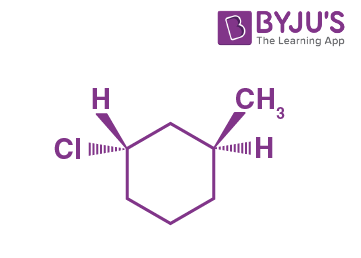Answer: The given compound has two chiral carbons having S configurations. Both of these carbons have S- configuration. Hence, the IUPAC name of the given compound is (1S, 3S)-1-chloro-3-methylcyclohexane.

Q6. Which intermediate has the highest energy?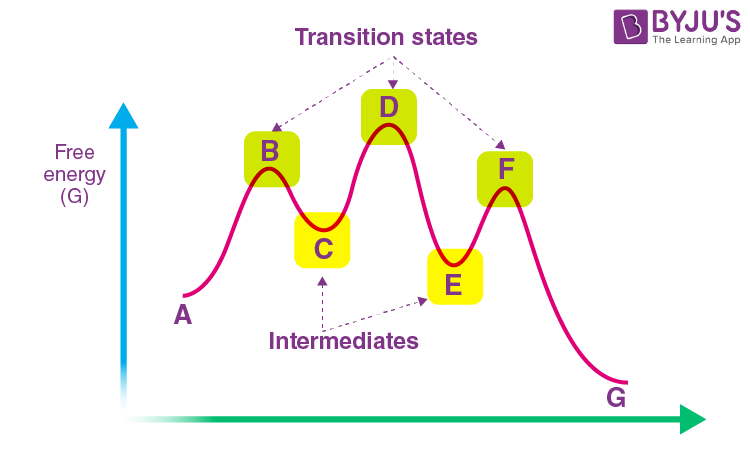Answer: The intermediate C has the highest energy. As the Ea required for the formation of C is much much higher than that in the case of E and the energy released as a result of formation of C is also very less.

Q7. What is the significance of adding H2SO4 during the addition of H2O to an alkene?

Answer: Since pure water is not acidic. Water is not capable of itself producing a strong enough electrophile (H+ ions) to initiate the reaction with the alkenes. Hence, sulphuric acid is mixed with water to make it acidic and to begin the reaction.

The addition of acidic water to an alkene leads to the formation of alcohols.

Q8. What happens when propene reacts with methanol in the presence of sulphuric acid?

Answer: Propene reacts with methanol in the presence of sulphuric acid to form 2-methoxypropane.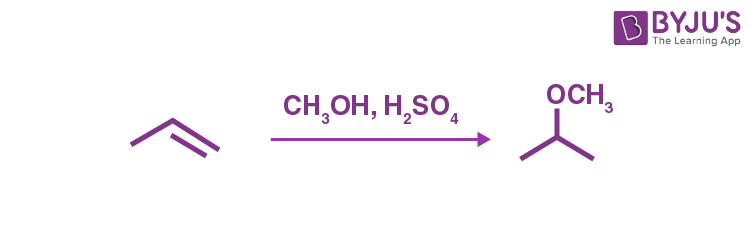Q9. Consider the given SN1 reaction:

(CH3)3C-Cl + I → (CH3)3C-I + Cl

What happens when the amount of I is doubled?

Answer: In the SN1 reactions, the nucleophile is not included in the rate determining step. Thus the rate does not change with the change in the nucleophile. Hence, no change occurs when the amount of I is doubled.

Q10. What is the relation between the given two structures?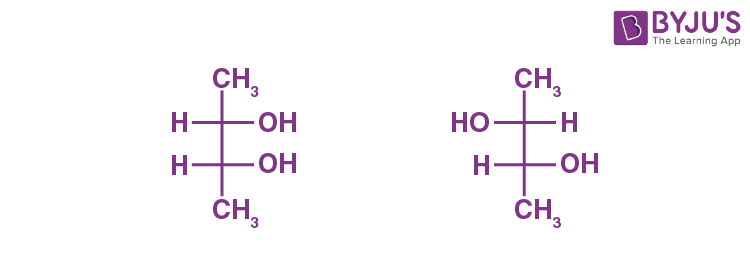Answer: The two given compounds are Diastereomers. Diastereomers are the non-superimposable, non-identical stereoisomers. They have the same molecular formula and same sequence of the bonding of atoms/ groups within the molecule but different spatial arrangement..

Q11. The specific rotation due to (R)-2-butanol is -13.5o. If the specific rotation of the mixture is -5.4o, what percentage of the mixture of the given two enantiomers will be the (S)-2-butanol?

Answer: In this case, the observed specific rotation is arising from the enantiomeric excess(e.e.). The percentage of e.e. can be calculated as:

% e.e. = [(-5.4o) / (-13.5o)] x 100 % = 40 %

Hence, (100 – 40) % = 60% of the remaining solution remains as the racemic mixture.

Thus, the total % of (R)-2-butanol = (40 + 60/2) % = 70%.

% of (S)-2-butanol = 100 % – 70 % = 30%.

Q12. Predict which of the following RX will undergo SN2 reaction most quickly?

1. CH3CH2F
2. CH3CH2I
3. CH3CH2Br
4. CH3CH2Cl

Explanation: During the Sn2 reactions, Nu attacks from the opposite of the leaving group. The easier and the faster the leaving group leaves, the quicker will be the addition of Nu. Among halogens, I ion is the best leaving group. Hence, CH3CH2I will react most quickly in SN2 reactions.

Q13. What is the relation between the given structures?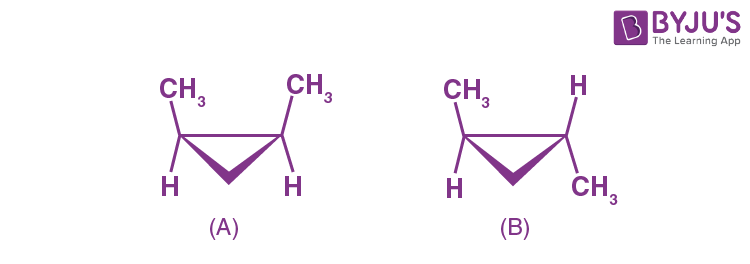1. Identical
2. Diastereomers
3. Structural isomers
4. Enantiomers

Explanation: Both the structures have the same molecular formula and the same sequence of bonded atoms/ groups. but they are non-identical and non-mirror images of each other. Hence, the two compounds are Diastereomers.

Q14. Which among the following shall undergo SN1 reaction most quickly?

1. CH3CH2CH2CH2Br
2. CH3CH2CH(CH3)Br
3. C(CH3)3Br

Explanation: The ease of the reaction depends on the stability of the carbocation formed as the reaction intermediate.

Q15. What is the driving force for the electrophilic addition reaction?

Answer: The driving force for the electrophilic addition reaction is the generation of an electrophile (E+) which attacks on the C=C. The E+ forms a covalent bond with the C=C leading to the formation of a carbocation.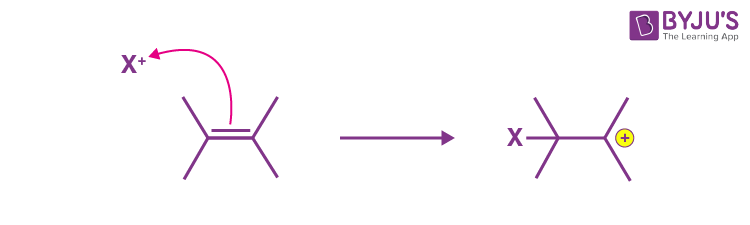The carbocation due to the positive charge density is then attacked by a nucleophile (Nu). The Nu forms another covalent bond with the carbocation.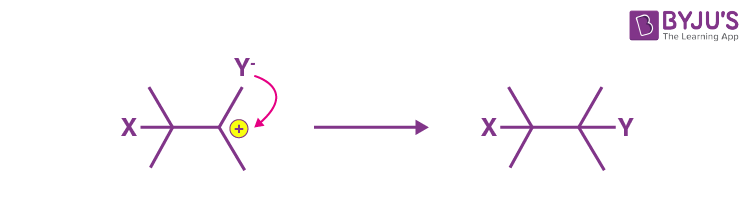Thus, in this process, 1 double bond breaks off and two new covalent bonds are formed.

## Practise Questions on Addition Reactions

Q1. Predict the major product of the following reaction:1.2.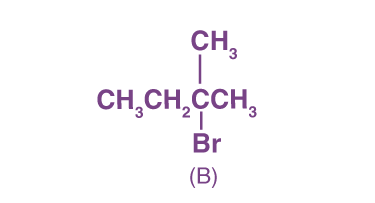3.4.Q2. Consider the given energy profile diagram. The rate of reaction depends on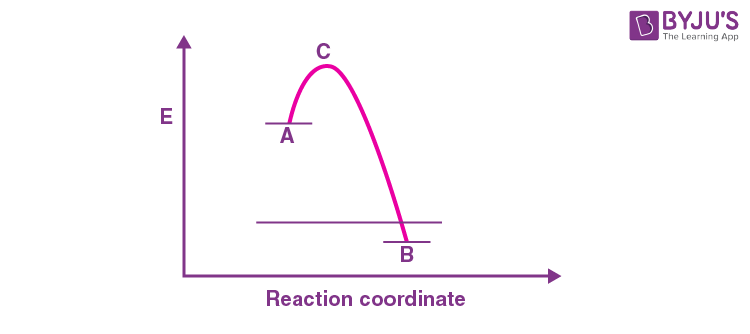1. The energy of A
2. The energy of B
3. The energy of C
4. The energy difference between A and C

Q3. Calculate ΔHo of the given reaction.

(CH3)3CH + Br2 → (CH3)3CBr + HBr

Given the bond dissociation energies (kcal/mol) are:

(H-Br) = 88 (kcal/mol)

(CH3)3C-H = 91 (kcal/mol)

(Br-Br) = 46 (kcal/mol)

(CH3)3C-Br = 65 (kcal/mol)

CH3-Br = 70 (kcal/mol)

Q4. Predict the sign of ΔSo for the reaction:

$$\begin{array}{l}CH_{2}=CH_{2} + H_{2} \xrightarrow[25\ ^{o}C]{catalyst}CH_{3}CH_{3}\end{array}$$

Q5. Which reactions are the reverse of Addition Reactions?

Click the PDF to check the answers for Practice Questions.

## Types of Organic Reactions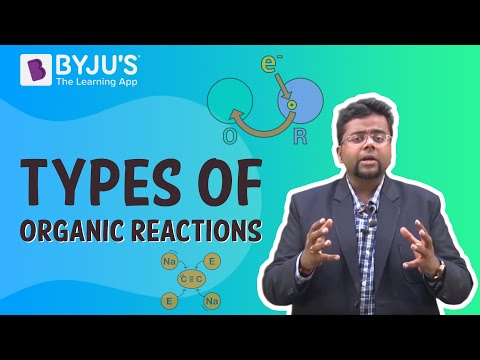## Chemical Reactions and Equations Class 10 Science